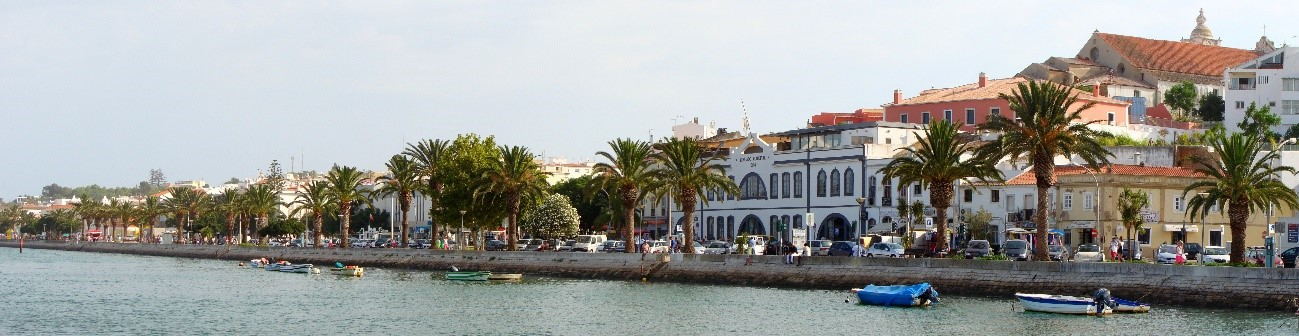## Eventos# Fifth International Workshop on Analysis and Numerical Approximation of Singular Problems (V IWANASP)

Qui, 22 Outubro 2015, 09:00 - Sab, 24 Outubro 2015, 19:00
Tipo(s) de Evento:
Workshop
Local do Evento:
Outro

The mathematical modelling of physical problems often leads to differential or integral equations whose coefficients, kernels, solutions etc. have singularities.

As in the previous IWANASP issues, the main objective of the present workshop is to bring togethermathematicians who deal with problems of this kind in different fields. The workshop will focus on the asymptotic properties of the solutions of equations and discretization methods.

As singular problems, we understand different kinds of  equations (including differential, integral, integro-differential and other types of functional equations) whose solutions have an unusual behaviour, which may assume the form of unboundedness, lack of smoothness, bifurcation, etc.

The topics that will be discussed include mathematical modelling of physical phenomena involving singularities; numerical solution of singular boundary value problems for differential equations; numerical integration of functions with singularities; computational methods for integral equations with singular kernels.

Applications in Biomedicine, Engineering, Finance, Insurance, are particularly welcome, both deterministic and stochastic.

Fifth International  Workshop on Analysis and Numerical Approximation of Singular Problems

Lagos 22-24 October